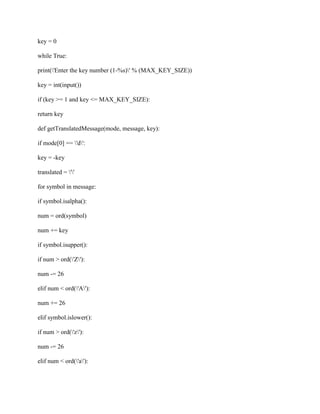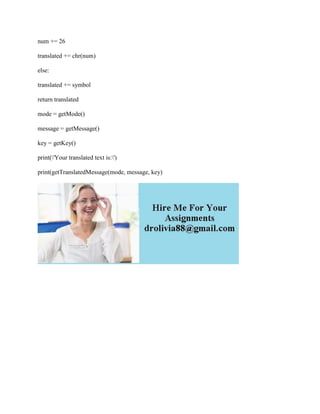Diese Präsentation wurde erfolgreich gemeldet.

# Develop a scheme program that encrypts characters but does not encrypt.docx

Anzeige
Anzeige
Anzeige
Anzeige
Anzeige
Anzeige
Anzeige
Anzeige
Anzeige
Anzeige
Anzeige×

1 von 3 Anzeige

# Develop a scheme program that encrypts characters but does not encrypt.docx

Develop a scheme program that encrypts characters but does not encrypt non-characters (example: ABC -> DEF) with 3 being used as the cipher key. Also develop the program to decipher your coded messages. You are asked to use lambda (ch) in your programs. Your program should read in plain text and write encrypted code on your screen.
Test data:
Joe
Solution
MAX_KEY_SIZE = 26
def getMode():
while True:
print(\'Do you wish to encrypt or decrypt a message?\')
mode = input().lower()
if mode in \'encrypt e decrypt d\'.split():
return mode
else:
print(\'Enter either \"encrypt\" or \"e\" or \"decrypt\" or \"d\".\')
def getMessage():
return input()
def getKey():
key = 0
while True:
print(\'Enter the key number (1-%s)\' % (MAX_KEY_SIZE))
key = int(input())
if (key >= 1 and key <= MAX_KEY_SIZE):
return key
def getTranslatedMessage(mode, message, key):
if mode == \'d\':
key = -key
translated = \'\'
for symbol in message:
if symbol.isalpha():
num = ord(symbol)
num += key
if symbol.isupper():
if num > ord(\'Z\'):
num -= 26
elif num < ord(\'A\'):
num += 26
elif symbol.islower():
if num > ord(\'z\'):
num -= 26
elif num < ord(\'a\'):
num += 26
translated += chr(num)
else:
translated += symbol
return translated
mode = getMode()
message = getMessage()
key = getKey()
print(getTranslatedMessage(mode, message, key)
.

Develop a scheme program that encrypts characters but does not encrypt non-characters (example: ABC -> DEF) with 3 being used as the cipher key. Also develop the program to decipher your coded messages. You are asked to use lambda (ch) in your programs. Your program should read in plain text and write encrypted code on your screen.
Test data:
Joe
Solution
MAX_KEY_SIZE = 26
def getMode():
while True:
print(\'Do you wish to encrypt or decrypt a message?\')
mode = input().lower()
if mode in \'encrypt e decrypt d\'.split():
return mode
else:
print(\'Enter either \"encrypt\" or \"e\" or \"decrypt\" or \"d\".\')
def getMessage():
return input()
def getKey():
key = 0
while True:
print(\'Enter the key number (1-%s)\' % (MAX_KEY_SIZE))
key = int(input())
if (key >= 1 and key <= MAX_KEY_SIZE):
return key
def getTranslatedMessage(mode, message, key):
if mode == \'d\':
key = -key
translated = \'\'
for symbol in message:
if symbol.isalpha():
num = ord(symbol)
num += key
if symbol.isupper():
if num > ord(\'Z\'):
num -= 26
elif num < ord(\'A\'):
num += 26
elif symbol.islower():
if num > ord(\'z\'):
num -= 26
elif num < ord(\'a\'):
num += 26
translated += chr(num)
else:
translated += symbol
return translated
mode = getMode()
message = getMessage()
key = getKey()